# How Make Cutest Hand Drawn Charts in Python

In this tutorial, I would like to introduce a very cool Python hand-painted style visualization package: cutecharts.

Different from the common charts such as Matplotlib and seaborn, this package can be used to generate the following kinds of charts that look like hand drawn, and the effect may be better in some scenarios. Cute charts are also interactive and dynamic. Whenever the mouse is hovering on the chart, the numbers show up.

For now, this library supports five kinds of charts — Bar, Line, Pie, Radar and Scatter. It also supports combination of charts. Let us explore them one by one.

## Import libraries and create data

Before we start to draw cute charts, we need to install the cutechart library.

``````pip install cutecharts
``````

``````import pandas as pd
import cutecharts.charts as ctc

df=pd.DataFrame({
'x':['Sun.','Mon.','Tue.','Wed.','Thu.','Fri.','Sat.'],
'y':[14,15,17,20,22.3,23.7,24.8],
'z':[16,16.4,23.6,24.5,19.9,13.6,13.4]})
``````

## Bar Chart

``````chart = ctc.Bar('Toronto Temperature', width='500px', height='400px')
chart.set_options(
labels=list(df['x']),
x_label='Days',
y_label='Temperature (Celsius)' ,
colors=['#1EAFAE' for i in range(len(df))]
)
chart.render()
``````hand drawn bar chart

Source code for this generated html file is here

## Line chart

It makes more sense to draw the line chart for our dataset so that we can see the differences between temperatures of last week and this week.

``````chart = ctc.Line('Toronto Temperature', width='500px', height='400px')
chart.set_options(
labels=list(df['x']),
x_label='Days',
y_label='Temperature (Celsius)'
)
chart.render()
``````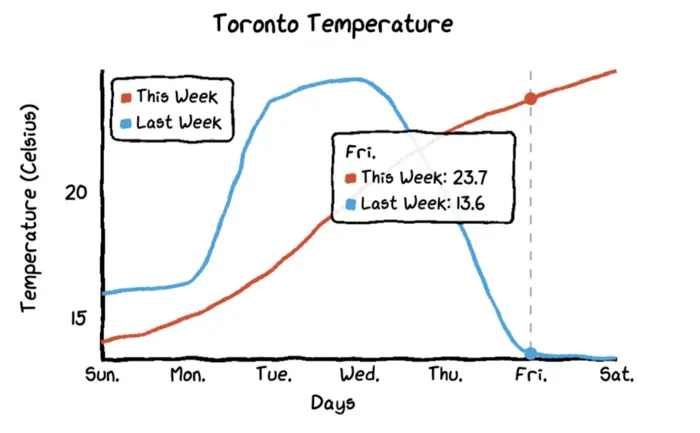hand drawn line chart

Source code for this generated html file is here

When you hover the mouse on the chart, the chart will automatically show labels with numbers and it also draws a dashed line so that the differences of temperatures between this week and last week become more visualized.

To change the line chart to a radar chart, you just need to change the chart type to ctc.Radar.

``````chart = ctc.Radar('Toronto Temperature', width='700px', height='600px')
chart.set_options(
labels=list(df['x']),
is_show_legend=True,    # by default, it is true. You can turn it off.
legend_pos='upRight'    # location of the legend
)
chart.render()
``````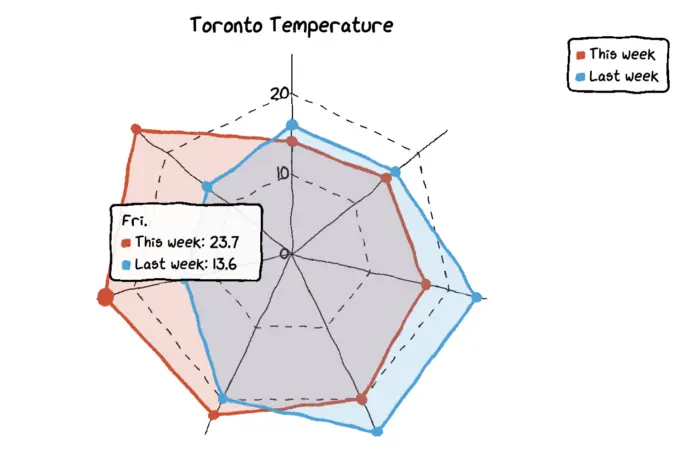hand drawn radar chart

Source code for this generated html file is here

## Pie chart

We need another dataset to make pie and donut charts. The datasets contains names of continents and their percentages of population.

``````df=pd.DataFrame({'x':['Asia', 'Africa', 'Europe', 'North America', 'South America', 'Australia'],
'y':[59.69, 16, 9.94, 7.79, 5.68, 0.54]})

chart = ctc.Pie('% of population by continent', width='1280px', height='720px')
chart.set_options(
labels=list(df['x']),
)
chart.render()
``````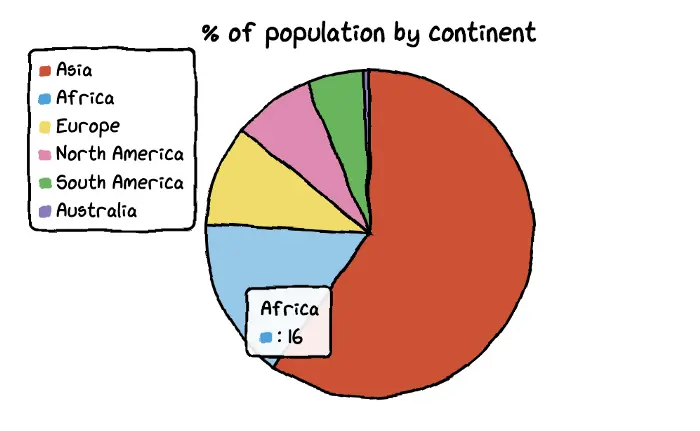hand drawn radar chart

Source code for this generated html file is here

And it is also very easy to turn a pie chart in to a donut chart. You just need to change the parameter of `inner_radius`.

``````df=pd.DataFrame({'x':['Asia', 'Africa', 'Europe', 'North America', 'South America', 'Australia'],
'y':[59.69, 16, 9.94, 7.79, 5.68, 0.54]})

chart = ctc.Pie('% of population by continent', width='1280px', height='720px')
chart.set_options(
labels=list(df['x']),
)
chart.render()
``````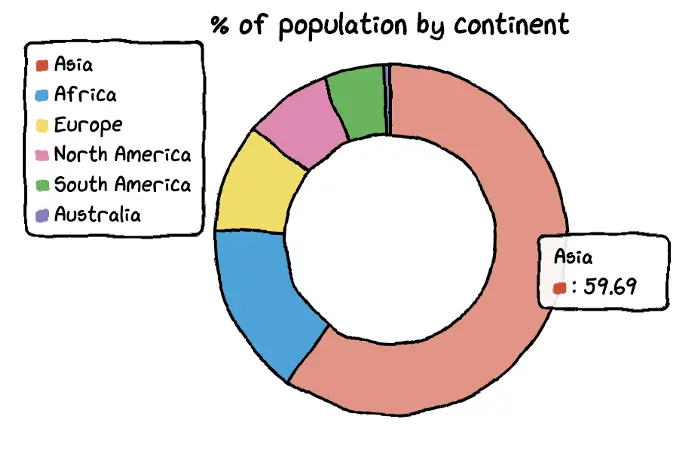hand drawn radar chart

Source code for this generated html file is here

## Scatter Plot

To plot the scatter plot, I will create a new dataset to map out the relationship between temperature and ice cream sales.

``````Temperature = [14.2, 16.4, 11.9, 15.2, 18.5, 22.1, 19.4, 25.1, 23.4, 18.1, 22.6, 17.2]
Sales       = [215, 325, 185, 332, 406, 522, 412, 614, 544, 421, 445, 408]

chart = ctc.Scatter('Ice Cream Sales vs Temperature', width='500px', height='600px')
chart.set_options(
x_label='Temperature (Celcius)',
y_label='Icecream Sales',
colors=['#1EAFAE'],
is_show_line = False,
dot_size=1
)
chart.add_series('Temperature', [(z, z) for z in zip(Temperature, Sales)])
chart.render()
``````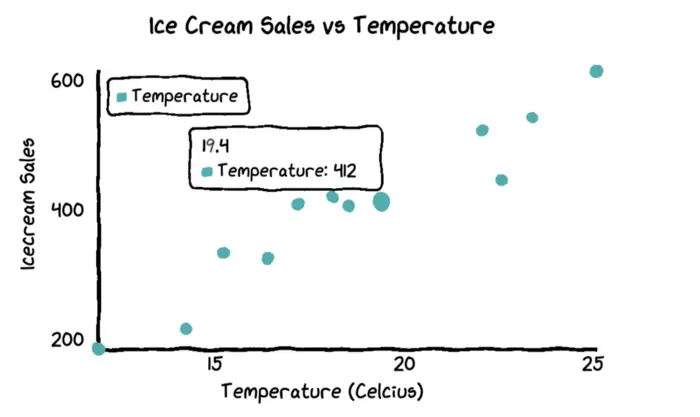hand drawn scatter plot

Source code for this generated html file is here

## Combined Charts

You are also able to combine multiple charts together.

``````import pandas as pd
import cutecharts.charts as ctc
from cutecharts.components import Page

df=pd.DataFrame({
'x':['Sun.','Mon.','Tue.','Wed.','Thu.','Fri.','Sat.'],
'y':[14,15,17,20,22.3,23.7,24.8],
'z':[16,16.4,23.6,24.5,19.9,13.6,13.4]})

chart1 = ctc.Line('Toronto Temperature', width='500px', height='400px')
chart1.set_options(
labels=list(df['x']),
x_label='Days',
y_label='Temperature (Celsius)'
)

chart2 = ctc.Bar('Toronto Temperature', width='500px', height='400px')
chart2.set_options(
labels=list(df['x']),
x_label='Days',
y_label='Temperature (Celsius)' ,
colors=['#1EAFAE' for i in range(len(df))]
)

page = Page()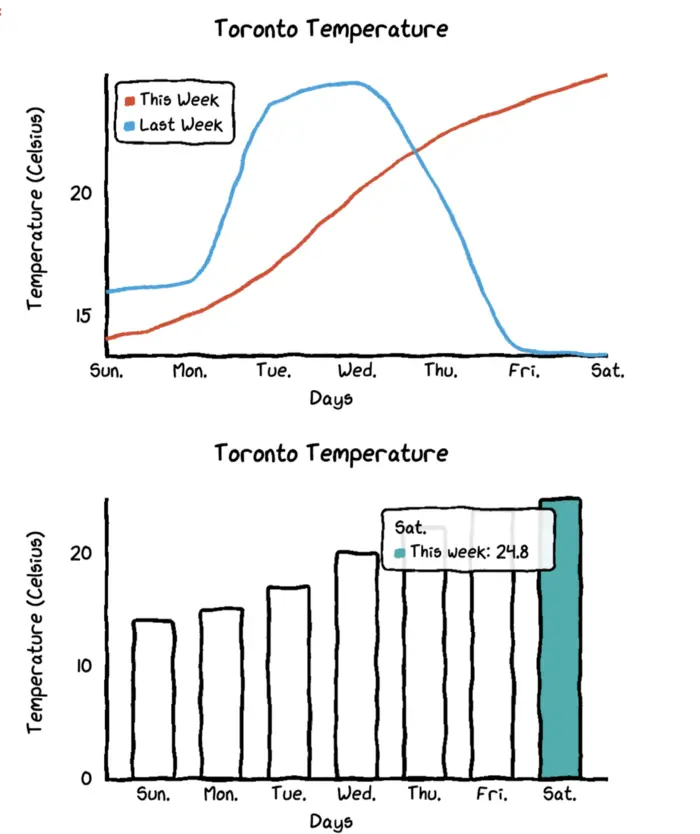hand drawn combined charts